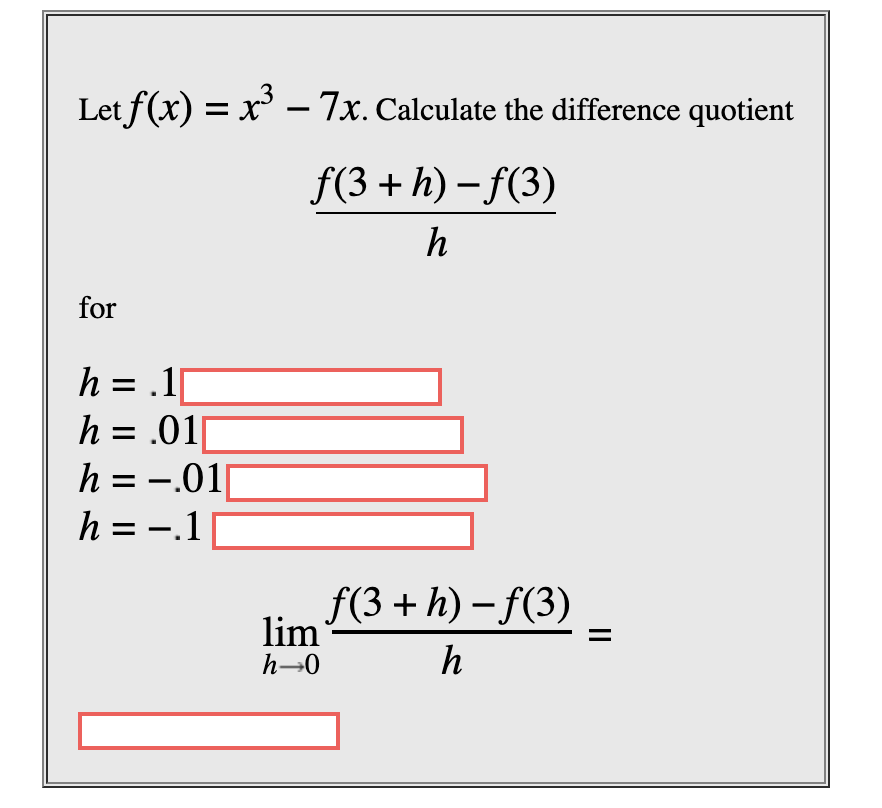# Let f(x)x3 -7x. Calculate the difference quotientf(3h)-(3)hforh .1h .01h -01=h -.1_f(3h)-f(3)limh-0h

Question
15 views

Let f(x)=x3-7x. Calculate the difference quotient

[f(3+h)-f(3)]/h

for

h=0.1

h=0.01

h=-0.01

h=-0.1help_outlineImage TranscriptioncloseLet f(x) x3 -7x. Calculate the difference quotient f(3h)-(3) h for h .1 h .01 h -01 = h -.1 _ f(3h)-f(3) lim h-0 h fullscreen
check_circle

star
star
star
star
star
1 Rating
Step 1

when h = 0.1,

Step 2

When h = 0.01

Step 3

When h = - ...

### Want to see the full answer?

See Solution

#### Want to see this answer and more?

Solutions are written by subject experts who are available 24/7. Questions are typically answered within 1 hour.*

See Solution
*Response times may vary by subject and question.
Tagged in